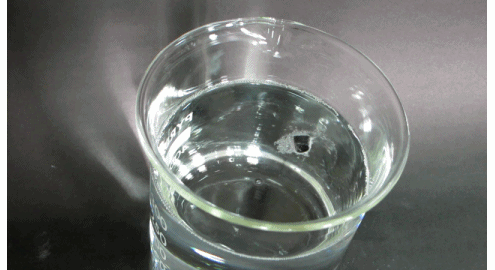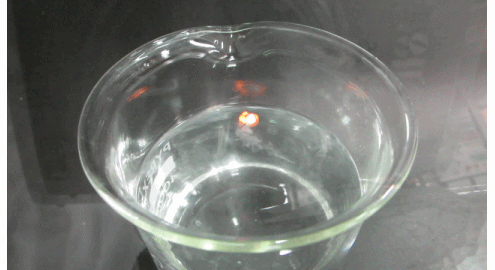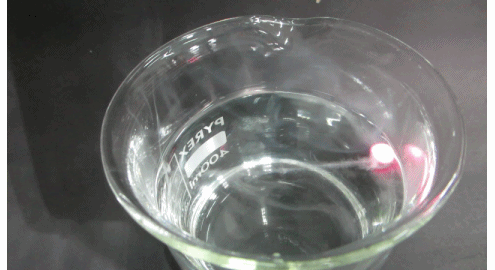# Reactions of Main Group Elements with Water

Water is composed of two hydrogen atoms and an oxygen atom. It exhibits polarity and is naturally found in the liquid, solid, and vapor states. Its polarity makes it a good solvent and is commonly known as the universal solvent. Because of its abundance on earth, it is important to note that it is involved in many chemical reactions. Many of these chemical reactions behave in trends that can be categorized using the periodic table.

## Group 1: Alkali Metals

A common characteristic of most Alkali Metals is their ability to displace H2(g) from water. This is represented by their large, negative electrode potentials. In this event, the Group 1 metal is oxidized to its metal ion and water is reduced to form hydrogen gas and hydroxide ions. The general reaction of an alkali metal (M) with H2O (l) is given in the following equation:

$\ce{ 2M(s) + 2H2O(l) \longrightarrow 2M^{+}(aq) + 2OH^{-}(aq) + H2 (g)}$

From this reaction it is apparent that OH- is produced, creating a basic or alkaline environment. Group 1 elements are called alkali metals because of their ability to displace H2(g) from water and create a basic solution.

Alkali metals are also known to react violently and explosively with water. This is because enough heat is given off during the exothermic reaction to ignite the H2(g).Figure $$\PageIndex{1}$$: Reactivity of Lithium (top), sodium (middle) and potassium (bottom) metals and water. (Free for Educational Use only, chemlegin).

### Alkali Metals Oxides and Water

Oxides of Group 1 elements also react with water to create basic solutions. Alkali metals react with oxygen to form monoxides, peroxides, or superoxides. These species react with water in different ways:

• Monoxides (M2O) produce alkali metal hydroxides:

$\ce{M2O(s) + 2H2O(l) \longrightarrow 2M^{+}(aq) + 2OH^{-} (aq)} \label{1}$

• Peroxides (M​2O2) produce metal hydroxides and hydrogen peroxide:

$\ce{M2O2(s) + 2H2O(l) \longrightarrow 2M^{+}(aq) + 2OH^{-} (aq) + H2O2(aq)} \label{2}$

• Superoxides (MO2) produce metal hydroxides, hydrogen peroxide, and oxygen gas:

$\ce{2MO2 (s) + 2H2O(l) \longrightarrow 2M^{+}(aq) + 2OH^{-} (aq) + H2O2 (aq) + O2(g)} \label{3}$

### Alkali Metal Hydrides and Water

Similarly to the Group 1 oxides, the hydrides of the Group 1 elements react with water to form a basic solution. In this case, however, hydrogen gas is produced with the metal hydroxide. The general reaction for alkali metal hydrides and water is given below:

$MH_{(s)} + H_2O_{(l)} \longrightarrow M^+_{(aq)}+OH^-_{(aq)}+H_{2(g)} \label{4}$

This reaction can be generalized to all alkali metal hydrides.

## Group 2: Alkaline Earth Metals

The majority of Alkaline Earth Metals also produce hydroxides when reacted with water. The hydroxides of calcium, strontium, and barium are only slightly soluble in water; however, enough hydroxide ions are produced to make a basic environment. The general reaction of calcium, strontium, and barium with water is represented below, where M represents calcium, strontium, or barium:

$M_{(s)} + 2H_2O_{(l)} \longrightarrow M(OH)_{2(aq)}+H_{2\;(g)} \label{5}$

Magnesium (Mg) reacts with water vapor to form magnesium hydroxide and hydrogen gas. Beryllium (Be) is the only alkaline earth metal that does not react with water. This is due to its small size and high ionization energy in relation to the other elements in the group.

### Alkaline Earth Metal Oxides and Water

Similarly to the alkali metal oxides, alkaline earth metal monoxides combine with water to form metal hydroxide salts (as illustrated in the equation below). The exception to this general assumption is beryllium, whose oxide (BeO) does not react with water.

$MO_{(s)}+H_2O_{(l)} \longrightarrow M(OH)_{2(s)} \label{6}$

One of the most familiar alkaline earth metal oxides is CaO or quicklime. This substance is often used to treat water and to remove harmful $$SO_{2(g)}$$ from industrial smokestacks.

### Alkaline Earth Metal Hydrides and Water

With the exception of beryllium (Be), the alkaline metal hydrides react with water to produce the metal hydroxide and hydrogen gas. The reaction of these metal hydrides can be described below:

$MH_{2(s)}+2H_2O_{(l)} \longrightarrow M(OH)_{2(aq)}+2H_{2(g)} \label{7}$

Hard Water

The two types of hard water include temporary hard water and permanent hard water. Temporary hard water contains bicarbonate (HCO3-) which forms CO3-2(aq), CO2(g), and H2O when heated. The bicarbonate ions react with alkaline earth cations and precipitate out of solution, causing boiler scale and problems in water heaters and plumbing. Common cations in the water include Mg+2 and Ca+2. In order to soften the water, water treatment plants add an alkaline earth metal hydroxide, such as slake lime [Ca(OH)2]. This solid dissolves in the water producing a metal ion (M+2) and hydroxide ions (OH-). The hydroxide ions combine with the bicarbonate ions in the water to produce water and a carbonate ion. The carbonate ion then precipitates out with the metal ion to form MCO3(s). Water treatment plants are able to remove the precipitated metal carbonate and thus soften the water.

The other type of hard water is permanent hard water. Permanent hard water contains bicarbonate ions (HCO3-) as well as other anions such as sulfate ions (SO4-2). The hardening species often cannot be boiled off. To soften permanent water, sodium carbonate (Na2CO3) is added. Sodium carbonate precipitates out the Mg+2 and Ca+2 ions out as the respective metal carbonates and introduces Na+ ions into the solutions.

## Group 13: Boron Family

Group 13 elements are not very reactive with water. In fact, boron (B) does not react at with water. One notable reaction within this group is aluminum's (Al) reaction with water. Aluminum does not appear to react with water because an outer layer of aluminum oxide (Al2O3) solid forms and protects the rest of the metal.

## Group 14: Carbon Family

For the most part, Group 14 elements do not react with water. One interesting consequence of this is that tin (Sn) is often sprayed as a protective layer on iron cans to prevent the can from corroding.Figure: An empty tin can. Steel cans are made of tinplate (tin-coated steel) or of tin-free steel. from Wikipedia.

## Group 15: Nitrogen Family

The pure elements in this family do not tend to react with water. Compounds of nitrogen (nitrates and nitrites) as well as nitrogen gas (N2) dissolve in water but do not react.

## Group 16: Oxygen Family

As mentioned earlier, many Group 1 and Group 2 oxides react with water to form metal hydroxides. The nonmetal oxides react with water to form oxoacids. Examples include phosphoric acid and sulfuric acid.

## Group 17: Halogens

Generally halogens react with water to give their halides and hypohalides. The halogen gases vary in their reactions with water due to their different electronegativities. Because fluorine ($$\ce{F2}$$) is so electronegative, it can displace oxygen gas from water. The products of this reaction include oxygen gas and hydrogen fluoride. The hydrogen halides react with water to form hydrohalic acids ($$\ce{HX}$$). With the exception of $$\ce{HF}$$, the hydrohalic acids are strong acids in water. Hydrochloric acid ($$\ce{HCl}$$), a strong acid, is an example.

$\ce{Cl2(g) + 2H2O(l) → HCl(aq) + HOCl(aq)}$

Hypochlorous ($$\ce{HOCl}$$) acid is a strong bleaching agent and is not very stable in solution and readily decomcomposes, especially when exposed to sunlight, yielding oxygen.

$\ce{ 2 HClO -> 2 HCl + O2}$

Bromine liquid dissolves slowly in water to form a yellowish-brown solution.

$\ce{Br2(g) + 2H2O(l) → HBr(aq) + HOBr(aq)}$

Hypobromous ($$\ce{HOBr}$$) acid is a weak bleaching agent.

$\ce{I2(g) + 2H2O(l) → HI(aq) + HOI(aq)}$

Only a little iodine dissolves in water to form a yellowish solution and hypoiodous ($$\ce{HOI}$$) acid has very weak bleaching characteristic.

## Group 18: Noble Gases

The noble gases do not react with water.

## General Summary

Reactant #1 Reactant #2 Products
Group 1 metal in period 3 or higher Cold Water Metal hydroxide & molecular hydrogen
Group 2 metal in period 3 or higher Cold Water Metal hydroxide & molecular hydrogen
Nonmetal element (excluding halogens) Cold Water No reaction
Fluorine (F2) Water Hydrogen fluoride (HF) and molecular oxygen (O2)
Halogen Water Hydrohalic acid or hypohalous acid
Metal with E0< -4.14 V for the lowest oxidation state Steam Metal oxide & molecular hydrogen
Nonmetal Halide Water Nonmetal oxide and hydrogen halide
Metal oxide Water Metal hydroxide
Nonmetal oxide Water oxoacid

## References

1. Birk, James P. "Predicting Inorganic Reactions." Expert System Applications in Chemistry. Washington: American Chemical Society, 1989. Print.
2. Huheey, James E., Ellen A. Keiter, and Richard L. Keiter. Inorganic Chemistry: Principles of Structure and Relativity. 4th ed. New York: HarperCollins College, 1993. Print.
3. Massey, A. G. Main Group Chemistry. London: Ellis Horwodd, 1990. Print.
4. Petrucci, et al. General Chemistry: Principles & Modern Applications. 9th ed. Upper Saddle River, New Jersey 2007.